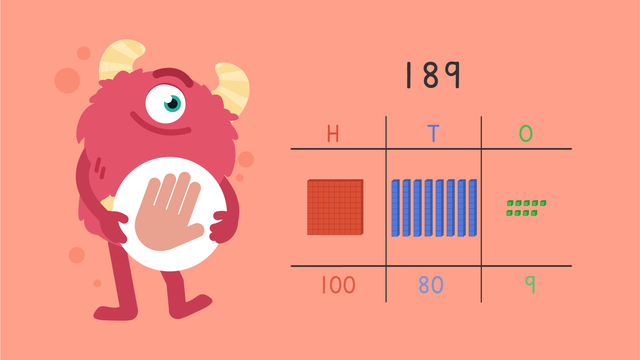# Expanded Form (3 Digits) — Let's Practice!Rating

Ø 4.5 / 2 ratings
The authorsTeam Digital
Expanded Form (3 Digits) — Let's Practice!
CCSS.MATH.CONTENT.2.NBT.A.3

## Basics on the topicExpanded Form (3 Digits) — Let's Practice!

Today we are practicing expanded form (3-digits) with Razzi! This video contains examples to help you further practice and grow confident in this topic.

### TranscriptExpanded Form (3 Digits) — Let's Practice!

Razzi says get these items ready, because today we're going to practice expanded form (three digits). It's time to begin! What is one hundred eighty-nine written in expanded form? There are one hundreds, eight tens, and nine ones. One hundred eighty-nine written in expanded form is one hundred plus eighty plus nine. Did you also get this answer? Let's tackle the next problem! What is four hundred twenty-six written in expanded form? There are four hundreds, two tens, and six ones. Four hundred twenty-six written in expanded form is four hundred plus twenty plus six. Did you also get this answer? Here is the next problem! What is three hundred fifty written in expanded form? There are three hundreds, five tens, and zero ones. Three hundred fifty written in expanded form is three hundred plus fifty plus zero. Did you also get this answer? Let's tackle the final problem! What is two hundred and eight written in expanded form? There are two hundreds, zero tens, and eight ones. Two hundred and eight written in expanded form is two hundred plus zero plus eight. Did you also get this? Razzi had so much fun practicing with you today! See you next time!

## Expanded Form (3 Digits) — Let's Practice! exercise

Would you like to apply the knowledge you’ve learned? You can review and practice it with the tasks for the video Expanded Form (3 Digits) — Let's Practice!.
• ### Choose the correct expanded form.

Hints

Say the number 873 out loud slowly. What did you hear? How many hundreds, tens, and ones?

Remember, all hundreds are followed by two zeros. For example: 100, 200, 300, 400, 500, 600, 700, 800, 900.

Remember, the tens place is a number followed by one zero. For example, the numbers 10, 20, or 30.

Look at the hundreds, tens, and ones in 873.

Solution

The correct expanded form for 873 is 800 + 70 + 3. This number had 8 hundreds (800), 7 tens (70), and 3 ones (3).

• ### Match each number correctly.

Hints

Remember, the expanded form shows the amount of hundreds, tens, and ones a number has.

Look at this example of expanded form:

700 + 60 + 2 = 762

Solution

All numbers expand to show the amount of hundreds, tens, and ones. When these hundreds, tens, and ones come together, we have a 3 digit number.

• ### What is 612 in expanded form?

Hints

Think about place value when writing the hundreds, tens, and ones.

Look at this example. 513 in expanded form is 500 + 10 + 3.

Solution

The expanded form for 612 is 600 + 10 + 2.

• ### What is this number?

Hints

Look at the number of hundreds, tens, and ones. Say them out loud.

If there were 3 hundreds, 5 tens, and 1 one, the number would be 351.

Solution

This number is 463. There are 4 hundreds (400), 6 tens (60), and 4 ones (4).

• ### Show the hundreds, tens, and ones.

Hints

Remember to give the hundreds, tens, and ones their appropriate place values. Example: 692 = 600 + 90 + 2

Count the hundreds out loud, then look for the matching number. Do the same with the tens and ones.

Solution

The number 384 has 3 hundreds, 8 tens, and 4 ones. The expanded form is: 300 + 80 + 4.

Hints